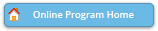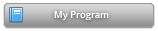#### Abstract Details

 Activity Number: 255 - Contributed Poster Presentations: Section on Statistical Computing Type: Contributed Date/Time: Monday, July 29, 2019 : 2:00 PM to 3:50 PM Sponsor: Section on Statistical Computing Abstract #305130 Title: Optimal Two-Stage Adaptive Subsampling Design for Softmax Regression Author(s): Yaqiong Yao* and HaiYing Wang and Jiahui Zou Companies: University of Connecticut and University of Connecticut and Academy of Mathematics and Systems Science, Chinese Academy of Sciences Keywords: Massive dataset; Optimal subsampling; Softmax regression; Optimality Criteria Abstract: For massive datasets, statistical analysis using the full data can be extremely time demanding and subsamples are often taken and analyzed according to available computing power. For this purpose, Wang et al. (2018) developed a novel two-stage subsampling design for logistic regression. We generalize this method to include the softmax regression, which has multiple categories for the responses. We derive the asymptotic distribution of the estimator obtained from subsamples that are drawn according to arbitrary subsampling probabilities, and then derive the optimal subsampling probabilities that minimize the asymptotic variance-covariance matrix under the A-optimality and the L-optimality criteria. The optimal subsampling probabilities involve unknown parameters, so we adopt the idea of optimal adaptive design and use a small subsample to obtain pilot estimators. In addition to subsampling with replacement, we also consider Poisson subsampling for its higher computational and estimation efficiency. We provide both simulation and real data examples to demonstrate the performance of our algorithm.

Authors who are presenting talks have a * after their name.